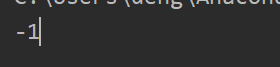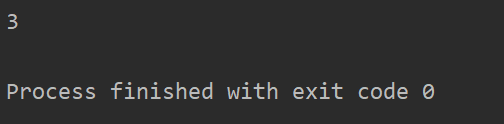• 最小： def getThreeNumberMin(x,y,z): min=x if x<y else y min=min if min<z else z return min a=getThreeNumberMin(3,-1,-1) print(a) 结果： 最大： def getThreeNumberMin(x,y,z): max=x if....
求最小：
def getThreeNumberMin(x,y,z):
min=x if x<y else y
min=min if min<z else z
return min
a=getThreeNumberMin(3,-1,-1)
print(a)


结果：求最大：
def getThreeNumberMin(x,y,z):
max=x if x>y else y
max=max if max>z else z
return max
a=getThreeNumberMin(3,3,-1)
print(a)展开全文• python 个数的均值import numpy as np np.mean([num1,num2,num n...])
python 求几个数的均值

import numpy as np
np.mean([num1,num2,num n...])
展开全文• 初学Python，会遇到各种各样的问题，小编也是在这样的...2.『成长』这词汇：其实对于Python的应用，我也是在探索的路上艰难前行，完全没达到敢"成长"这词的地步，共同努力。啰嗦完毕，进入正题。...
初学Python，会遇到各种各样的问题，小编也是在这样的过程中成长起来的。以上是我想说的第一句话，那么对于我的上一句话，有两点需要声明：1.『小编』这个称谓：第一次写文章，实在不知道从哪开始，只是之前看到大家都自称"小编"，所以，觉得这是一个普遍的称谓吧。2.『成长』这个词汇：其实对于Python的应用，我也是在探索的路上艰难前行，完全没达到敢用"成长"这个词的地步，共同努力。啰嗦完毕，进入正题。###############################学习任何一门编程语言，使用任何一门语言进行编程，想要实现一个功能或是解决一个问题，方法都不止一个，这是恒不变的规律。那么，每次遇到问题的时候，我都会查阅几种方法进行对比，然后择其优者而从之，尽管多数都需要改动才能用，但人家的方法总之给了你灵感或点化的作用。所以，在解决问题的过程中，一定要有自己的判断。###############################小编在一次解决问题的过程中，遇到了比较三个数大小的问题，然后在探索的过程中，在网上发现一个方法，而且赞同的人还挺多的，但反复梳理它的流程，发现总是有些问题，然后拿来经过数据验证，找出了一些问题，下面直接上代码。一。引用的方法：def max_min(x, y, z):
max = min = x
if y > max:
max = y
else:
min = y
if z > max:
max = z
else:
min = z
return (max, min)
print(max_min( 15,10,39))这个方法的问题在于，如果传参是这样的，print(max_min(39，10，15))，它的结果是max=39，min=15，结果显然是不对的。分析其原因，是考虑到了大数的提取，没考虑到有一种情况下，两个小数没有进行比较。二，改进后的代码：c=[]
def max_min(x,y,z):
max = min = x
if y > max:
max = y
if z > max:
max = z
else:
if z < min:
min = z
else:
min = y
if z > max:
max = z
else:
if z < min:
min = z
return(max,min)

c.append(max_min(1,2,3))

print(c)
print(c,c)那么，问题来了，这样的方法就是好的吗？答案是否定的，首先，从它的长度上来看，就觉得复杂，尽管它经过验证是对的。既然是学习，就要举一反三、触类旁通。所以在后面我真正使用的过程中，我还是用了其他的方法，我用的是列表的方式解决的。以下是代码：三。用列表排序解决比大小问题：def max_min(x, y, z):
list = []
list.append(x)
list.append(y)
list.append(z)
list.sort()
return list
print(max_min(x, y, z))好了，我不知道它是不是最优的方案，但它完美解决了我的问题。所以我决定不再修改它。如果有同学看到这篇分享，欢迎提出您的宝贵建议和意见。如果这些方法解决了您的问题，那么，深感荣幸！！！#################################纪念一下自己的第一次分享，期待更多。。。
展开全文• ## python三个数之和

千次阅读 2018-07-13 18:25:56
def find_threedigit(arr, target): arr.sort() result = [] ...最简单的计算三个数为某个的方法， 思路是两层循环，固定其中的两个，那么剩下的一个也固定了，很简单的方法，大家应该可以理解
def find_threedigit(arr, target):
arr.sort()
result = []
for i in range(len(arr)-1):
if arr > target:
return -1

for j in range(i+1, len(arr)):
temp = arr[i] + arr[j]
if temp <= target and ((target - temp) in arr[j::]):
print(result.append([arr[i], arr[j], target - temp]))

return result

if __name__ == "__main__":
arr = list(map(int, input().split()))
target = int(input())  #测试输入的时候，写的是12，下面的结果为12 的结果
print(find_threedigit(arr, target))out：2 3 4 5 6 7 12
[[2, 3, 7], [2, 4, 6], [2, 5, 5], [3, 4, 5]]今天只来讨论一种最简单的计算三个数之和为某个数的方法，思路是两层循环，固定其中的两个数，那么剩下的一个数也固定了，很简单的方法，大家应该可以理解
展开全文• ## Python求取逆序数

千次阅读 2021-03-18 10:09:29
Python求取逆序方法一.py方法二.py方法.py 方法一.py #利用简单的数学计算 num = int(input('请输入一个三位正整数：')) a = num//100 b = num%100//10 c = num%100%10 print('该的反序为：',(100*c+10*b+a)...算法
• ## Python求水仙花数

千次阅读 2018-08-19 13:11:10
• @demo小计 python求所有中的水仙花 for i in range(100, 1000): a = int(str(i)[-1]) b = int(str(i)[-2]) c = int(str(i)) if a ** 3 + b ** 3 + c ** 3 == i: print(i) 结果
• 最后比较products1products2，返回得出较大乘积的三个数。 2、乘积最小的三个数： 先出列表中最小的三个数的乘积 products1； 再出列表中最大的二个与最小的之乘积 products2； 最后比较products...
• python三个数从小到大排序 1、首先定义一个函数paiLie()；然后在paiLie函数内使用for循环input获取三个数字并存入列表；最后调用列表的sort()方法进行排序即可。 def paiLie(): result = [] for i in range(3): ...人工智能 编程语言 机器学习 java
• 若能整除这三个数，则输出其中的最小的即为最小公倍数。 代码如下： def Sort_xyz(x,y,z,): l = [x,y,z] l.sort() return l def Gcd(x,y,z): x,y,z = Sort_xyz(x,y,z) for i in range(1,z + 1...
• python作业7-如果一正整数等于除它本身之外其他所有除数之，就称之为完全。 例如：6是完全， 因为6 = 1+2+3； 下一完全是28 = 14+7+4+2+1。 1000以下的完全 打印出1000以下的完全 打印出1000以下...
• b=[1,2,3,4]print(b)for i in range(len(b)): for j in range(len(b)): for k in range(len(b)): if b[i]!=b[j] and b[i] != b[k] and b[j] !=b[k]: print(b[i],b[j],b[k]) ...
• ## python求三角形面积

万次阅读 2018-11-02 10:59:52
Python第一课 目前正在自学python,上来做点笔记。...题目：已知三条边，如何三角形的面积? 在这里插入代码片 `import math a=4.0 b=3.0 c=5.0 d=(a+b+c)/2 s=math.sqrt(d*(d-a)*(d-b)*(d-c)) print (s) ...
• a = int(input("请输入a的值:"))#Input录入的是字符，但我们使用的是整形，所以要类型转换一下 b = int(input("请输入b的值:")) c = int(input("请输入c的值:")) if(a ): max = b min = a else: max = a min = b if...
• 原理：将一个数等分后，各等分后的的乘积最大。 如果该无法等分，就将余数加到任意一等分的上。 Python方法实现： &gt;&gt;&gt; def max_product(sum): mod = sum % 3 a = b = (sum - mod)...
• ## Python求完全数

千次阅读 2019-11-23 22:03:08
完全（Perfect number），又称完美或完备，是一些特殊的自然数。...第一个完全是6，第二个完全是28，第三个完全是496，后面的完全还有8128、33550336等等。 for n in range(2,1000): sum=0 fo...
• 定义一个三 a = 123456789 百位数： a//100%10 十位： a//10%10 位数： a//1%10 取倒数第几位，就是a//位数%10
• #输入三个数，输出这三个数的最大值。 a = input() b = input() c = input() if a > b and a > c: print(a) elif b > c : print(b) else: print(c)
•  Python——无序数组中找出为N的两个三个数、四个） 【问题描述】无序数组中找出为N的两个，例如，nums = [1, 4, 3, 2, 6, 5]中找出为target = 6的序列，答案：[(1, 5), (4, 2)]。 参考博客： 1、...
• num3 = float(input('请输入第三个数：')) if num1 &amp;amp;amp;lt; num3 and num2 &amp;amp;amp;lt; num3: big_num = num3 elif num1 &amp;amp;amp;lt; num2 and num3 &amp;am
• 没有任何编程基础的菜鸟，初学Python，写的一个求三个数的最小公倍数的代码。 做法是先找出较大的那个的倍数，再这个除以其余看能否被整除，不满足的话继续计算较大的二倍，三倍，直到满足能被其余两个...算法
• python四个中取三个数组成互不相同且没有重复数字的三位 问：有1，2，3，4四个数字，能够组成多少个互不相同而且无重复数字的三位？都是多少？ 最简单就是下面的这种： nums=[] for i in range(1,5): for j ...
• ## Python求黑洞数

万次阅读 2019-04-29 09:49:59
# 五位数及其以上的黑洞不只一，而是几数字循环出现，这里就不了 下面的代码是通过递归获取一数字的黑洞 def black_hole_Number ( number , pre_number_list = None ) : number = str ( ...
• 水仙花是指一N位正整数（N≥3），它的每位上的数字的N次幂之等于它本身。 例如：153=1×1×1+5×5×5+3×3×3。 本题要求编写程序,计算所有N位水仙花。 输入格式: 输入在一行中给出一正整数N（3≤N≤5）...
• python小程序(2)#输入三个整数，将这三个数由小到大输出 思路： 1.先将a,b两个做比较，并将较大的放在第一的位置，较小的放在第二的位置。 2.再将c分别与第一的与第二的作比较，这里分了三种情况： c最大，将...程序
• #前40斐波那契 #方法1 L=[] a=0 b=1 n=0 while n&lt;40: L+=[b] a,b=b,a+b n+=1 print("前四十斐波那契有：",L) print("----------------") #方法2 L=[] for s in range(40):...
• x=int(input("请输入第一个："...请输入第三个数：")) if x&gt;y: if x&gt;z: print('x是最大值:%d'%x) else: print('z是最大值:%d'%z) elif x&gt;z: if x&gt;y: ...
• 时间：2018-10-29概述：水仙花数Python打印出所有的“水仙花”，所谓“水仙花”是指一个三，其各位数字立方等于该本身。例如：153是一“水仙花”，因为153=1的次方＋5的次方＋3的次方。编写...
• 题目：正整数的最大公约数最小公倍数。 基本要求：1.程序风格良好(使用自定义注释模板)，两种以上算法解决最大公约数问题，提供友好的输入输出。 提高要求： 1.种以上算法解决两正整数最大公约数问题......

# 如何用python求三个数的和python 订阅